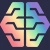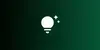PromptBaseExplore
ModelsMidjourneyDALL·EStable DiffusionGPTArt & IllustrationsAnimeCartoonPaintingIllustrationUnique StylesLogos & IconsLogoIconGraphics & DesignPatternProduct DesignProfile PictureProductivity & WritingProductivityWritingCodingMarketing & BusinessMarketingBusinessSocial MediaPhotographyPhotographyPhotography StyleGames & 3D3DFun & GamesVideo Game ArtGeneral
Home
Marketplace
Generate
Hire
Sell
🤖 GPT# Knowledge Tutor - Any Field Of Study1Favorites
60Views
TestedTipsCHAT GPT transforms into your personal tutor and provides a comprehensive lesson plan for any subject you want to learn bout. 👨‍🎓📕 This prompt can find its use upon professors of any subject in making a study curve for their students or for people who want to learn subjects they have little to no knowledge about.👀🚀 In case you need any aditional changes to the original prompt send me a private message and I will do my best to alter it to your specific needs with no aditional charge. 🤙👊🏻
...more
\$3.99
9 months ago

#### Prompt Details

Model
Chat - GPT-3.5 (gpt-3.5-turbo)
Token size
158 (\$0.0003 / call)
Example input
Subject: Geometry
Example output
Lesson Plan: Introduction to Geometry Goal: To introduce the fundamental concepts of geometry to a beginner student and provide a solid foundation for future studies in the subject. Duration: This lesson plan is designed to be completed in 1-2 weeks, depending on the pace of the student. Materials: Notebook or paper Pencil or pen Ruler Compass (optional) Lesson 1: Points, Lines, and Angles Introduce the basic elements of geometry: points, lines, and angles. Define a point as a location in space with no size or shape. Define a line as a straight path that extends infinitely in both directions. Define an angle as the measure of the space between two intersecting lines or line segments. Demonstrate how to draw a line and an angle using a ruler and a protractor (if available). Have the student draw several points, lines, and angles in their notebook. Exercise 1: Draw three different points, connect them with three different lines, and draw three different angles using a protractor (if available). Lesson 2: Polygons and Circles Introduce the concepts of polygons and circles. Define a polygon as a closed shape made up of straight line segments. Define a circle as a closed shape in which all points on the perimeter are equidistant from the center. Demonstrate how to draw a polygon and a circle using a ruler and a compass (if available). Discuss the properties of polygons, such as the number of sides, angles, and vertices. Discuss the properties of circles, such as the radius, diameter, and circumference. Exercise 2: Draw a triangle, a square, a pentagon, and a hexagon, and label the number of sides, angles, and vertices for each. Draw a circle, label the radius, diameter, and circumference, and draw an inscribed and a circumscribed polygon. Lesson 3: Congruence and Similarity Introduce the concepts of congruence and similarity. Define congruence as the property of having the same size and shape. Define similarity as the property of having the same shape but not necessarily the same size. Discuss how to determine if two figures are congruent or similar. Introduce the concept of transformations, such as translation, rotation, and reflection, and how they affect congruence and similarity. Exercise 3: Draw two triangles that are congruent and two triangles that are similar, and label the corresponding sides and angles. Perform a translation, rotation, and reflection on a polygon, and draw the resulting image. Lesson 4: Pythagorean Theorem and Trigonometry Introduce the Pythagorean Theorem and how it relates to right triangles. Define the Pythagorean Theorem as a² + b² = c², where a and b are the legs of a right triangle and c is the hypotenuse. Demonstrate how to use the Pythagorean Theorem to find the length of a missing side of a right triangle. Introduce the concept of trigonometry and the three basic trigonometric functions: sine, cosine, and tangent. Discuss how to use trigonometry to solve for missing sides and angles of right triangles. Exercise 4: Given a right triangle with legs of length 3 and 4, find the length of the hypotenuse using the Pythagorean Theorem. Given a right triangle with an angle of 30 degrees and a hypotenuse of 5, find the length of the adjacent and opposite sides using trigonometry. Additional Resources: Khan Academy's Geometry course: The Geometry section on MathIsFun.com The book "Geometry Essentials for Dummies" by Mark Ryan The online platform GeoGebra for interactive geometry explorations Past papers and sample questions for geometry exams and competitions such as the SAT, ACT, and AMC Anticipated Questions and Misconceptions: Q: What is the difference between a line segment and a line? A: A line is an infinite path that extends infinitely in both directions, while a line segment is a finite portion of a line with two endpoints. Q: What is the difference between a regular polygon and an irregular polygon? A: A regular polygon is a polygon with all sides and angles congruent, while an irregular polygon has sides and angles of different lengths and measures. Q: How do I know if two triangles are similar? A: Two triangles are similar if their corresponding angles are congruent and their corresponding sides are proportional. Q: What is the difference between sine, cosine, and tangent? A: Sine, cosine, and tangent are trigonometric functions that relate the ratios of the sides of a right triangle to its angles. Sine is the ratio of the opposite side to the hypotenuse, cosine is the ratio of the adjacent side to the hypotenuse, and tangent is the ratio of the opposite side to the adjacent side. Misconception: All circles have the same size. Correction: The size of a circle is determined by its radius or diameter, and different circles can have different sizes. Misconception: All polygons are similar. Correction: Two polygons are similar if they have the same shape but not necessarily the same size. Some polygons, such as a square and a rectangle, can be similar but not congruent. Conclusion: This lesson plan provides a comprehensive introduction to the fundamental concepts of geometry for a beginner student. By building a solid foundation of knowledge, the student will be prepared for future studies in the subject and be able to apply the concepts learned to solve real-world problems. With a mix of theory and practical exercises, the student will have a well-rounded understanding of geometry and be equipped with the skills and knowledge to continue their exploration of the subject.